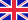•# Mandelbrot Set

Autor: Frederic P. Miller

In mathematics the Mandelbrot set, named after Benoît Mandelbrot, is a set of points in the complex plane, the boundary of which forms a fractal. Mathematically the Mandelbrot set can be defined as the set of complex values of c for which the orbit of 0... Viac o knihe

Na objednávku, dodanie 2-4 týždne

29.75 €

bežná cena: 35.00 €

## O knihe

In mathematics the Mandelbrot set, named after Benoît Mandelbrot, is a set of points in the complex plane, the boundary of which forms a fractal. Mathematically the Mandelbrot set can be defined as the set of complex values of c for which the orbit of 0 under iteration of the complex quadratic polynomial zn+1 = zn2 + c remains bounded. That is, a complex number, c, is in the Mandelbrot set if, when starting with z0=0 and applying the iteration repeatedly, the absolute value of zn never exceeds a certain number (that number depends on c) however large n gets. For example, letting c = 1 gives the sequence 0, 1, 2, 5, 26,..., which tends to infinity. As this sequence is unbounded, 1 is not an element of the Mandelbrot set. On the other hand, c = i (where i is the square root of -1) gives the sequence 0, i, (-1 + i), -i, (-1 + i), -i..., which is bounded and so i belongs to the Mandelbrot set. When computed and graphed on the complex plane the Mandelbrot Set is seen to have an elaborate boundary which does not simplify at any given magnification.

• Vydavateľstvo: Alphascript Publishing
• Rok vydania: 2009
• Formát: Paperback
• Rozmer: 220 x 150 mm
• Jazyk: Anglický jazyk
• ISBN: 9786130240233

Generuje redakčný systém BUXUS CMS spoločnosti ui42.